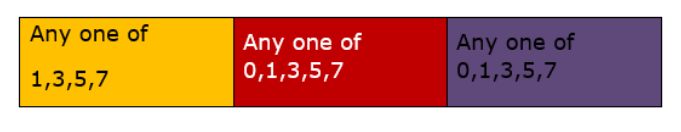# How many 3-digit numbers can be formed by using the digits

Question:

How many 3-digit numbers can be formed by using the digits 0, 1, 3, 5, 7 while each digit may be repeated any number of times?

Solution:

100’s place 10’s place Unit’s placeThere are total 5 digits available, for forming a 3 digit number, in 100's place only $1,3,5,7$ can be used( 0 not included because it will lead to formation of 2 digit number.) In 10 's place any of the 5 can be used and same is the case with one's place.

So total number of 3 digit numbers formed $=4 \times 5 \times 5=100$